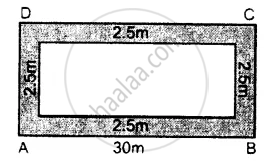# A Path of Uniform Width, 2.5 M, Runs Around the Inside of a Rectangular Field 30 M by 27 M. Find the Area of the Path. - Mathematics

Sum

A path of uniform width, 2.5 m, runs around the inside of a rectangular field 30 m by 27 m. Find the area of the path.

#### Solution

According to the given statement, the figure will be as shown alongside.Clearly, the length of the rectangular field including the path = 30 m.
Its Area = 30 x 27 = 810 m2
Width of the path = 2.5 m
Length of the rectangular field including the path = 30 – 2.5 – 2.5 = 25 m.
Breadth = 27 – 2.5 – 2.5 = 22m
Area of the rectangular field including the path = 25 x 22 = 550 m2
Hence, area of the path = 810 – 550 = 260 m2.

Is there an error in this question or solution?
Chapter 20: Area of a Trapezium and a Polygon - Exercise 20 (B) [Page 227]

#### APPEARS IN

Selina Concise Mathematics Class 8 ICSE
Chapter 20 Area of a Trapezium and a Polygon
Exercise 20 (B) | Q 15 | Page 227
Share